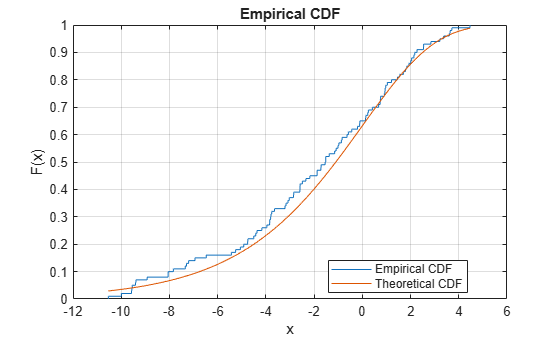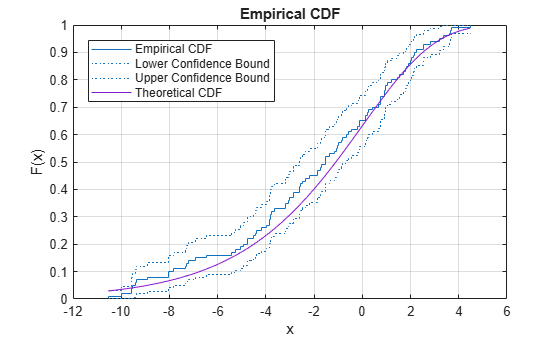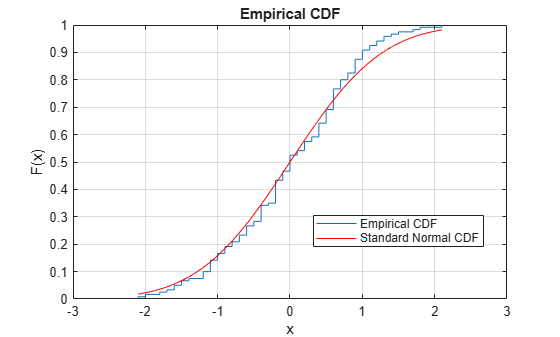cdfplot

Empirical cumulative distribution function (cdf) plot

Description

example

cdfplot(x) creates an empirical cumulative distribution function (cdf) plot for the data in x. For a value t in x, the empirical cdf F(t) is the proportion of the values in x less than or equal to t.

h = cdfplot(x) returns a handle of the empirical cdf plot line object. Use h to query or modify properties of the object after you create it. For a list of properties, see Line Properties.

[h,stats] = cdfplot(x) also returns a structure including summary statistics for the data in x.

Examples

collapse all

Plot the empirical cdf of a sample data set and compare it to the theoretical cdf of the underlying distribution of the sample data set. In practice, a theoretical cdf can be unknown.

Generate a random sample data set from the extreme value distribution with a location parameter of 0 and a scale parameter of 3.

rng('default')  % For reproducibility
y = evrnd(0,3,100,1);

Plot the empirical cdf of the sample data set and the theoretical cdf on the same figure.

cdfplot(y)
hold on
x = linspace(min(y),max(y));
plot(x,evcdf(x,0,3))
legend('Empirical CDF','Theoretical CDF','Location','best')
hold offThe plot shows the similarity between the empirical cdf and the theoretical cdf.

Alternatively, you can use the ecdf function. The ecdf function also plots the 95% confidence intervals estimated by using Greenwood's Formula. For details, see Algorithms.

ecdf(y,'Bounds','on')
hold on
plot(x,evcdf(x,0,3))
grid on
title('Empirical CDF')
legend('Empirical CDF','Lower Confidence Bound','Upper Confidence Bound','Theoretical CDF','Location','best')
hold offPerform the one-sample Kolmogorov-Smirnov test by using kstest. Confirm the test decision by visually comparing the empirical cumulative distribution function (cdf) to the standard normal cdf.

Load the examgrades data set. Create a vector containing the first column of the exam grade data.

Test the null hypothesis that the data comes from a normal distribution with a mean of 75 and a standard deviation of 10. Use these parameters to center and scale each element of the data vector, because kstest tests for a standard normal distribution by default.

x = (test1-75)/10;
h = kstest(x)
h = logical
0

The returned value of h = 0 indicates that kstest fails to reject the null hypothesis at the default 5% significance level.

Plot the empirical cdf and the standard normal cdf for a visual comparison.

cdfplot(x)
hold on
x_values = linspace(min(x),max(x));
plot(x_values,normcdf(x_values,0,1),'r-')
legend('Empirical CDF','Standard Normal CDF','Location','best')The figure shows the similarity between the empirical cdf of the centered and scaled data vector and the cdf of the standard normal distribution.

Input Arguments

collapse all

Input data, specified as a numeric vector.

Data Types: single | double

Output Arguments

collapse all

Handle of the empirical cdf plot line object, returned as a chart line object. Use h to query or modify properties of the object after you create it. For a list of properties, see Line Properties.

Summary statistics for the data in x, returned as a structure with the following fields:

FieldDescription

min

Minimum value

max

Maximum value

mean

Sample mean

median

Sample median (50th percentile)

std

Sample standard deviation

Tips

• cdfplot is useful for examining the distribution of a sample data set. You can overlay a theoretical cdf on the same plot of cdfplot to compare the empirical distribution of the sample to the theoretical distribution. For an example, see Compare Empirical cdf to Theoretical cdf.

• The kstest, kstest2, and lillietest functions compute test statistics derived from an empirical cdf. cdfplot is useful in helping you to understand the output from these functions. For an example, see Test for Standard Normal Distribution.

Alternative Functionality

You can use the ecdf function to find the empirical cdf values and create an empirical cdf plot. The ecdf function enables you to indicate censored data and compute the confidence bounds for the estimated cdf values.